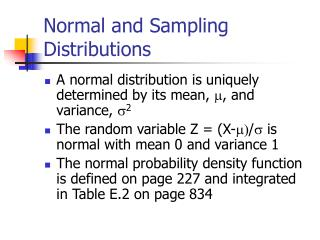DownloadDownload PresentationNormal and Sampling Distributions

# Normal and Sampling Distributions

Download Presentation## Normal and Sampling Distributions

- - - - - - - - - - - - - - - - - - - - - - - - - - - E N D - - - - - - - - - - - - - - - - - - - - - - - - - - -
##### Presentation Transcript

1. Normal and Sampling Distributions • A normal distribution is uniquely determined by its mean, m, and variance, s2 • The random variable Z = (X-m)/s is normal with mean 0 and variance 1 • The normal probability density function is defined on page 227 and integrated in Table E.2 on page 834

2. Sampling Distributions • A sampling distribution is the probability distribution of a random variable that is a sample statistic • Sample mean • Sample proportion • Sample standard deviation • Sample correlation coefficient

3. Central Limit Theorem • The sampling distribution of the sample mean is approximately normal • The larger the sample size, n, the more closely the sampling distribution of the sample mean will resemble a normal distribution.

4. The Sampling Distribution of the Sample Mean • Mean = m, the same as the mean of X • Variance = s2/n, the variance of X divided by sample size

5. The Sampling Distribution of the Sample Proportion • Mean = p, the population proportion of or the probability of success in the binomial trial • Variance = p(1-p)/n. • The binomial distribution is approximately normal if np and n(1-p) are both at least 5.